# The cost 3

The cost of 2 ¾ litres of petrol is Rs 250 ½. What is the cost of 1 litre of petrol?

c =  91.0909

### Step-by-step explanation: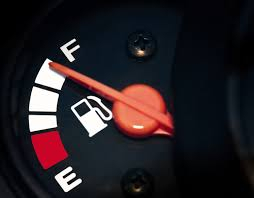Did you find an error or inaccuracy? Feel free to write us. Thank you!Tips to related online calculators
Tip: Our volume units converter will help you with the conversion of volume units.

## Related math problems and questions:

• There 14There are 250 people in a museum. 2/5 of the 250 people are girls 3/10 of the 250 people are boys The rest of the 250 people are adults Work out the number of adults in the museum.
• Gas, lpg and diesel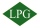Calculate how much it costs to drive 35000 km by car: a) a car with a consumption of 8l/100 km of LPG for 60 cents b) 7l/100 km of petrol for 1.33 euros c) 6 l/100 km of diesel for 1.2 euros. Calculate what is cheaper.
• Pen and pencil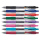Cost of a pen is Rs. 12 3/5 and cost of a pencil is Rs. 3 2/5. What is the total cost of pen and pencil?
• WaterMix 68 l of water with a temperature of 87 °C, 17 l warm of 42 °C, and 55 l water of 50 °C. What is the temperature of the mixed water immediately after mixing?
• A box 2A box has a length of 4 1/2 inches, a width of 3 2/3 inches and a height of 8 1/4 inches. What is the volume of the box?
• A bottle 2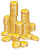A bottle contain 255 coins 1/3 of the coins are £1 cons 110 of the coins are 50p coins the rest of the coins are 20p coins. What is the total value of the coins contained in the bottle.
• What is 9What is the value of x in the proportion 2 and one-fourth over x = 1 and one-half over 3 and three-fifths? 2 and two-fifths 5 and two-fifths 8 and 1 over 10 12 and 3 over 20
• Gasoline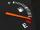For nearly full tank of 40 liters of gasoline chauffeur paid 54 euros. How many euros it will cost to go 250 km long if the car has an average fuel consumption 7 liters per 100 kilometers?
• Simplify expression with mixedWhen (7¹/3 + 2¾)÷(2 + 2¼ × 1¹/3) is simplified the result is?
• Fractions and mixed numerals(a) Convert the following mixed numbers to improper fractions. i. 3 5/8 ii. 7 7/6 (b) Convert the following improper fraction to mixed number. i. 13/4 ii. 78/5 (c) Simplify these fractions to their lowest terms. i. 36/42 ii. 27/45 2. evaluate following ex
• RatiosReduce the numbers: 50 in a 1:2 ratio 111 at a ratio of 2:3 70 at 10:50 560 at a ratio of 3:8
• Volume per time 2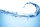Benjamin drinks tea at an incredible rate. He drinks 3 1/2 liters of tea every 2/3 of an hour. Ben drinks tea at a constant rate. How much does he drink in one hour?
• ZarkaZarka purchased 3 1/2 meters of cloth for 100 3/8 euros. How much does 1 meter of cloth cost?
• Car consumption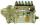The car has consumption 4.4 l/100 km. How much money will it cost ride 450 km in long if diesel price is 1.72 USD/l?
• Kilo candyOne kilogram of chocolate candy cost 10 euros. Kate buys 250 grams and had the 8 pack of candy. How much does cost one bonbon?
• ErikThree friends Peter, Erik and Milan shared 420 euros in a ratio of 1: 2: 3. How many euros did Erik get?
• The product 2The product of two functions is 10. If one of them is 2 1/3, find the other one.